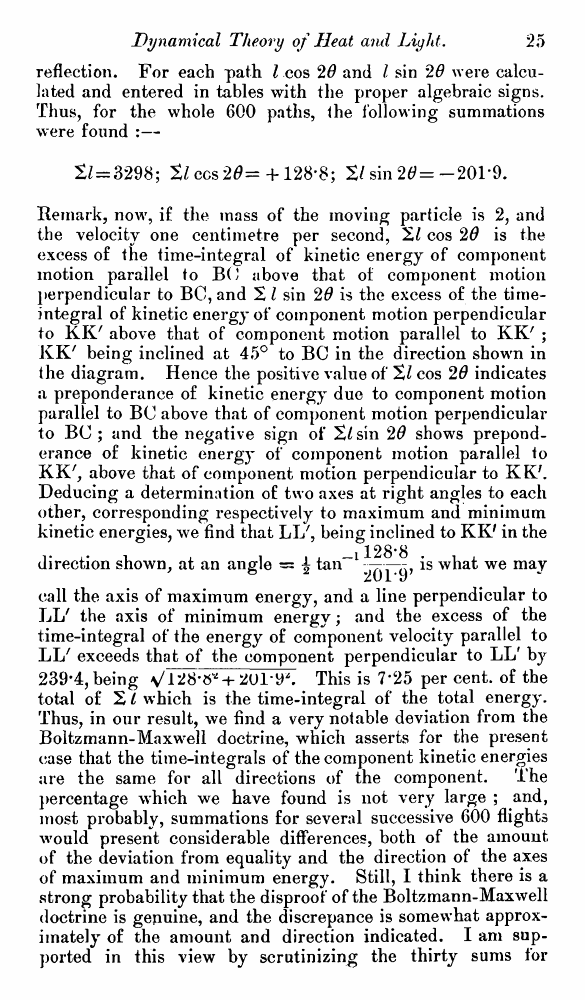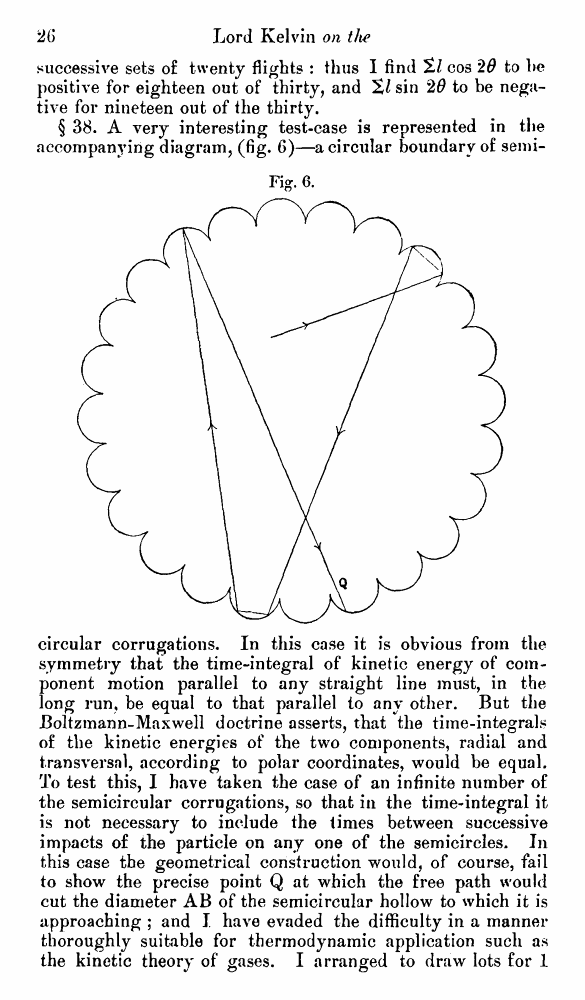﻿ ﻿ Lord Kelvin. Nineteenth-Century Clouds over the Dynamical Theory of Heat and Light. // Phil. Mag. S. 6. Vol. 2. No. 7. July 1901.

# Lord Kelvin. Nineteenth-Century Clouds over the Dynamical Theory of Heat and Light. // Phil. Mag. S. 6. Vol. 2. No. 7. July 1901.

В начало   Другие форматы   <<<     Страница 25   >>>1  2  3  4  5  6  7  8  9  10  11  12  13  14  15  16  17  18  19  20  21  22  23  24  25 26  27  28  29  30  31  32  33  34  35  36  37  38  39  40 reflection. For each path I cos 20 and I sin 20 were calculated and entered in tables with the proper algebraic signs. Thus, for the whole 600 paths, the following summations were found :— 2/ = 3298; tl ccs 20= + 128*8; 2/sin 20=-201'9. Remark, now, if the mass of the moving particle is 2, and the velocity one centimetre per second, 2/ cos 20 is the excess of the time-integral of kinetic energy of component motion parallel to BO above that of component motion perpendicular to BC, and 2 I sin 20 is the excess of the time-integral of kinetic energy of component motion perpendicular to KK7 above that of component motion parallel to KK' ; KK' being inclined at 45° to BC in the direction shown in the diagram. Hence the positive value of 21 cos 20 indicates a preponderance of kinetic energy due to component motion parallel to BC above that of component motion perpendicular to BC ; and the negative sign of 2/sin 20 shows preponderance of kinetic energy of component motion parallel to KK', above that of component motion perpendicular to KK/. Deducing a determination of two axes at right angles to each other, corresponding respectively to maximum and minimum kinetic energies, we find that LI/, being inclined to KK' in the 128*8 direction shown, at an angle = \ tan“l ^j—is what we may call the axis of maximum energy, and a line perpendicular to LL' the axis of minimum energy; and the excess of the time-integral of the energy of component velocity parallel to LL' exceeds that of the component perpendicular to LL' by 239-4, being s/1+ 2U1-9*. This is 7*25 per cent, of the total of 2/ which is the time-integral of the total energy. Thus, in our result, we find a very notable deviation from the Boltzmann-Maxwell doctrine, which asserts for the present case that the time-integrals of the component kinetic energies are the same for all directions of the component. The percentage which we have found is not very large ; and, most probably, summations for several successive 600 flights would present considerable differences, both of the amount of the deviation from equality and the direction of the axes of maximum and minimum energy. Still, I think there is a strong probability that the disproof of the Boltzmann-Maxwell doctrine is genuine, and the discrepance is somewhat approximately of the amount and direction indicated. I am supported in this view by scrutinizing the thirty sums for successive sets of twenty flights : thus I find cos 29 to he positive for eighteen out of thirty, and %l sin 20 to be negative for nineteen out of the thirty. § 38. A very interesting test-case is represented in the accompanying diagram, (fig. 6)—a circular boundary of semi- Fig. 6. circular corrugations. In this case it is obvious from the symmetry that the time-integral of kinetic energy of component motion parallel to any straight line must, in the long run, be equal to that parallel to any other. But the Boltzmann-Maxwell doctrine asserts, that the time-integrals of the kinetic energies of the two components, radial and transversal, according to polar coordinates, would be equal. To test this, I have taken the case of an infinite number of the semicircular corrugations, so that in the time-integral it is not necessary to include the times between successive impacts of the particle on any one of the semicircles. In this case the geometrical construction would, of course, fail to show the precise point Q at which the free path would cut the diameter AB of the semicircular hollow to which it is approaching ; and I have evaded the difficulty in a manner thoroughly suitable for thermodynamic application such as the kinetic theory of gases. I arranged to draw lots for 1